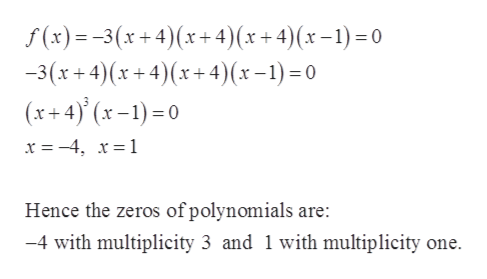# Fidn the zeros of the polynomial function, and state the multiplictity of each. f(x)= -3(x+4)(x+4)(x+4)(x-1)

Question

Fidn the zeros of the polynomial function, and state the multiplictity of each.

f(x)= -3(x+4)(x+4)(x+4)(x-1)

check_circle

Step 1

Given:

Step 2

To find zeros of given po...help_outlineImage Transcriptionclosef(x)3(x+4) (x+ 4)(x + 4)(x-1)=0 3(x+4)(x+4) (x+ 4)(x-1)=0 (x+4)(x-)0 x = -4, x= 1 Hence the zeros of polynomials are: 4 with multiplicity 3 and 1 with multiplicity one fullscreen

### Want to see the full answer?

See Solution

#### Want to see this answer and more?

Solutions are written by subject experts who are available 24/7. Questions are typically answered within 1 hour.*

See Solution
*Response times may vary by subject and question.
Tagged in

### Algebra